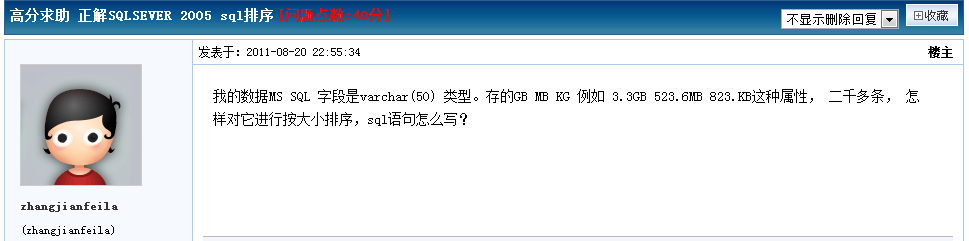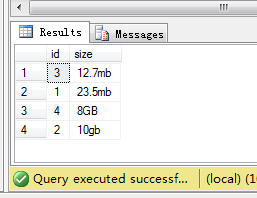# --如果长度少于等于2的数值为返回NULL，下面利用sql函数存储过程来实现的一种大小排序的方法

• 栏目：编程 时间：2020-04-25 23:39
<返回列表

SET ANSI_NULLS ON GO SET QUOTED_IDENTIFIER ON GO ALTER FUNCTION [dbo].[udf_OrderLimitSize] ( @Ov NVARCHAR(30) ) RETURNS decimal(18,6) AS BEGIN --如果长度少于等于2的数值为返回NULL IF (LEN(@Ov) = 2) RETURN NULL --宣告两个变量 DECLARE @v DECIMAL(18,6),@n DECIMAL(18,6)

--判断最后两位数是否为下面这些单位 IF (RIGHT(@Ov,2) NOT IN ('TB','GB','MB','KB')) RETURN NULL --去掉最后两位数之后，把值转为DECIMAL数据类型 SET @n = CONVERT(DECIMAL(18,6),LEFT(@Ov, LEN(@Ov) - 2)) --判断截除最后两位数之后，使用ISNUMERIC判断是否为有效的数值，如果不是返回NULL IF (ISNUMERIC(@n) = 0) RETURN NULL --下面做单位转算，如果遇上有新单位时，可以作相应添加 IF (@Ov LIKE '%TB') SET @v = @n * 1024 * 1024 * 1024 IF (@Ov LIKE '%GB') SET @v = @n * 1024 * 1024 IF (@Ov LIKE '%MB') SET @v = @n * 1024 IF (@Ov LIKE '%KB') SET @v = @n RETURN @v ENDCREATE TABLE test(id int identity(1,1),size NVARCHAR(50)) GO INSERT INTO [test] values('23.5mb'),('10gb'),('12.7mb'),('8GB') go SELECT [id],[size] FROM test ORDER BY [dbo].[udf_OrderLimitSize]([size])udf_OrderLimitSize

SET ANSI_NULLS ON
GO
SET QUOTED_IDENTIFIER ON
GO
ALTER FUNCTION [dbo].[udf_OrderLimitSize]
(
@Ov NVARCHAR(30)
)
RETURNS decimal(18,6)
AS
BEGIN

--如果长度少于等于2的数值为返回NULL
IF (LEN(@Ov) <= 2)
RETURN NULL

--宣告两个变量
DECLARE @v DECIMAL(18,6),@n DECIMAL(18,6)

--判断最后两位数是否为下面这些单位
IF (RIGHT(@Ov,2) NOT IN ('TB','GB','MB','KB'))
RETURN NULL

--去掉最后两位数之后，把值转为DECIMAL数据类型
SET @n = CONVERT(DECIMAL(18,6),LEFT(@Ov, LEN(@Ov) - 2))

--判断截除最后两位数之后，使用ISNUMERIC判断是否为有效的数值，如果不是返回NULL
IF (ISNUMERIC(@n) = 0)
RETURN NULL

--下面做单位转算，如果遇上有新单位时，可以作相应添加
IF (@Ov LIKE '%TB')
SET @v = @n * 1024 * 1024 * 1024
IF (@Ov LIKE '%GB')
SET @v = @n * 1024 * 1024
IF (@Ov LIKE '%MB')
SET @v = @n * 1024
IF (@Ov LIKE '%KB')
SET @v = @n
RETURN @v
END

CREATE TABLE test(id int identity(1,1),size NVARCHAR(50))
GO
INSERT INTO [test] values('23.5mb'),('10gb'),('12.7mb'),('8GB')
go
SELECT [id],[size] FROM test ORDER BY [dbo].[udf_OrderLimitSize]([size])# 更多阅读

## 只能填一个，// 接口密码\$code =

\$loginname = ; // 接口帐号\$pass = ; // 接口密码\$code = ;\$subid =;\$sender = ;\$receivers = ; // 接收号码，多个...

## 完全可以在程序内调用外部命令

PHP作为一种服务器端的脚本语言，象编写简单，或者是复杂的动态网页这样的任务，它完全能...